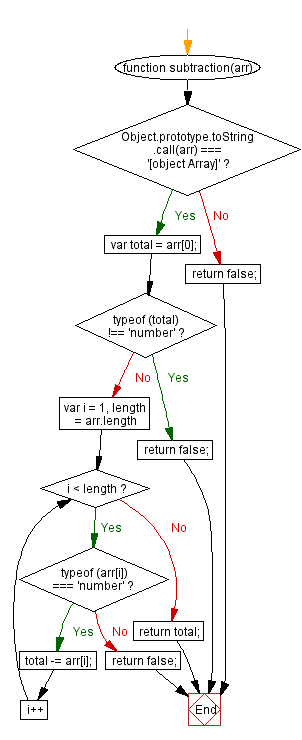# JavaScript: Subtract elements from one another in an array

## JavaScript Math: Exercise-45 with Solution

Write a JavaScript function to subtract elements from an array.

Sample Solution:-

HTML Code:

``````<!DOCTYPE html>
<html>
<meta charset="utf-8">
<title>JavaScript function to subtract elements from one another in array</title>
<body>

</body>
</html>
```
```

JavaScript Code:

``````function subtraction(arr)
{
if (Object.prototype.toString.call(arr) === '[object Array]') {
var total = arr;
if (typeof (total) !== 'number') {
return false;
}
for (var i = 1, length = arr.length; i < length; i++)
{
if (typeof (arr[i]) === 'number')
{
total -= arr[i];
}
else
return false;
}
}
else
return false;
}
console.log(subtraction([7,3, 2,-1]));
```
```

Sample Output:

```3
```

Pictorial Presentation:Flowchart:Live Demo:

See the Pen javascript-math-exercise-45 by w3resource (@w3resource) on CodePen.

Improve this sample solution and post your code through Disqus

What is the difficulty level of this exercise?

Test your Programming skills with w3resource's quiz.

﻿